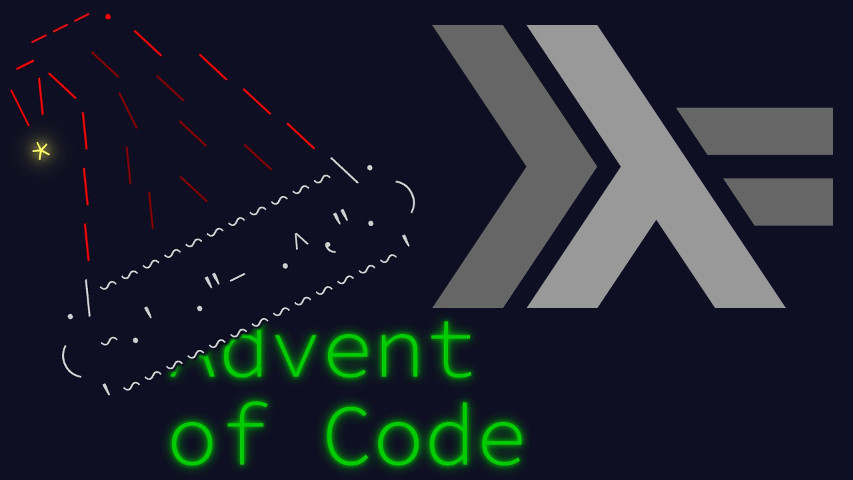AoC Day 8: Seven Segment Search2021-12-08T21:18:52+01:00

For today’s Advent of Code, “Seven Segment Search”, we’re given a jumbled wiring of seven segment displays, the span of possible observations, and the one we actually want to decode. This post is your usual literate Haskell, with the obligatory imports prologue.

``````import Control.Arrow ((&&&))
import Data.List     (elemIndex,find,permutations,sort)
import Data.Maybe    (fromJust)``````

We are given the input as segments observed. To make our life easier, let’s represent them as integers 0 to 6 instead of letters a to g:

``````type Segment = Int
newtype Observation = Observation { view :: [Segment] }``````

Parsing an input line is a simple matter of converting from alphabetic to numeric:

``````parse :: String -> ([Observation],[Observation])
parse l = (digits,display) where
(digits,_:display) = splitAt 10 (map readObservation (words l))

An observation is a one-on-one mapping of segments to wires activated. The central system that commands the wires only activates them in patterns of valid digits.

``````type Wire = Int
newtype Digit = Digit [Wire] deriving (Eq,Ord)``````

We are given the valid patterns. To be able to compare them with one another, I’ll sort them before wrapping in the `Digit` newtype.

``````combine :: Observation -> Digit
combine = Digit . sort . view

reference :: [Digit]
reference = map (combine . readObservation)
[ "abcefg", "cf", "acdeg", "acdfg", "bcdf"
, "abdfg", "abdefg", "acf", "abcdefg", "abcdfg"
]``````

Solving an input line is now a matter of finding which original digit an observation matches.

Intelligent solving would have us write a table of first-order logic equations and reduce it by various propagation and/or algebraic algorithms.

But the search space is small enough (7! = 5040) that we can just iterate over it until we find a match…

``````solve :: [Observation] -> [Observation] -> [Int]
solve obsDigits obsDisplay =
let permute p = map (combine . Observation . map (p !!) . view)
Just perm = find ((== sort reference) . sort . flip permute obsDigits)
(permutations [0..6])``````

…and apply the singled out permutation to look up the digits in our reference table.

``  in map (fromJust . (`elemIndex` reference)) (permute perm obsDisplay)``

Part 1 asks for the number of digits that have a unique number of activated segments. We get it by solving the scramble and looking them up.

Part 2 asks for the sum of displayed numbers. We get it by solving the scramble, decoding decimal and summing.

``````main :: IO ()
main = interact \$ show .
( length . filter (`elem` [1,4,7,8]) . concat &&&
sum . map (foldl (\a b -> 10*a + b) 0) ) .
map (uncurry solve . parse) . lines``````

It’s obvious this problem was written so as to be solvable by deduction, for example:

• 1 is the unique 2-segment digit
• 7 is the unique 3-segment digit
• 4 is the unique 4-segment digit
• 8 is the unique 7-segment digit
• 6 is the 6-segment digit that does not include 1
• 9 is the 6-segment digit that includes 4
• 0 is the remaining 6-segment digit
• 3 is the 5-segment digit that includes 1
• 5 is the 5-segment digit that’s included in 6
• 2 is the remaining 5-segment digit

Yet when search is so accessible… why bother?

This concludes today’s solution. See you soon!